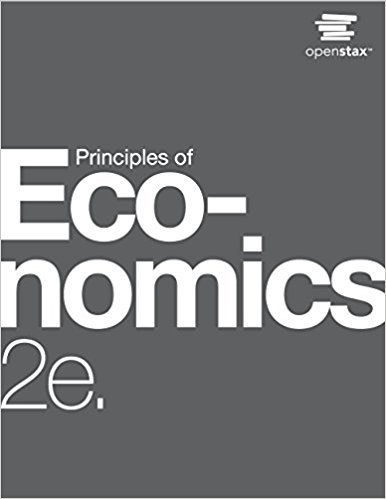×
Get Full Access to Principles Of Economics - 2 Edition - Chapter 7 - Problem 14
Get Full Access to Principles Of Economics - 2 Edition - Chapter 7 - Problem 14

×

# How do we calculate marginal productISBN: 9781947172364 471

## Solution for problem 14 Chapter 7

Principles of Economics | 2nd Edition

• Textbook Solutions
• 2901 Step-by-step solutions solved by professors and subject experts
• Get 24/7 help from StudySoup virtual teaching assistantsPrinciples of Economics | 2nd Edition

4 5 1 407 Reviews
20
3
Problem 14

How do we calculate marginal product?

Step-by-Step Solution:
Step 1 of 3

d"4'fu-/ Z i ,B,r^A*r,S-,gsir- t2/z/r- .j,-. tlrp o.k/;h 4seqn-f /sr Et-Lca,,+-

Step 2 of 3

Step 3 of 3

##### ISBN: 9781947172364

This textbook survival guide was created for the textbook: Principles of Economics, edition: 2. Since the solution to 14 from 7 chapter was answered, more than 230 students have viewed the full step-by-step answer. This full solution covers the following key subjects: . This expansive textbook survival guide covers 37 chapters, and 1291 solutions. The answer to “How do we calculate marginal product?” is broken down into a number of easy to follow steps, and 6 words. Principles of Economics was written by and is associated to the ISBN: 9781947172364. The full step-by-step solution to problem: 14 from chapter: 7 was answered by , our top Business solution expert on 03/16/18, 04:24PM.

Unlock Textbook Solution